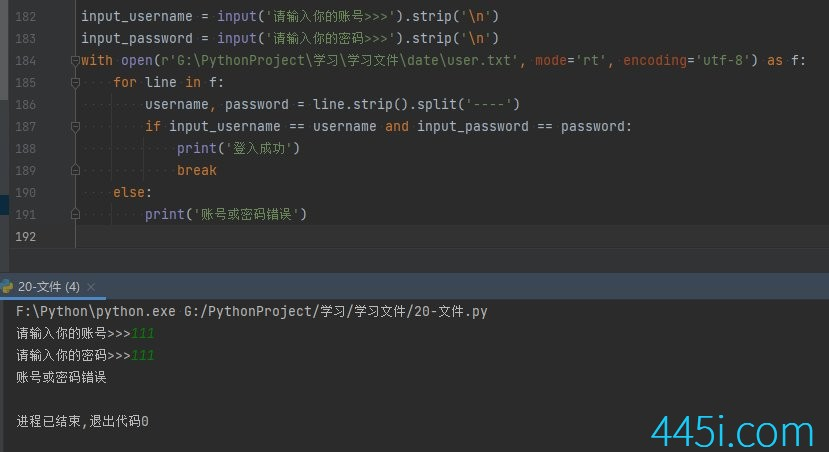# Python 多账号登入案例

``````with open(r'G:\PythonProject\学习\学习文件\date\user.txt', mode='rt', encoding='utf-8') as f:
for line in f:
l = line.strip().split('----')``````

``````with open(r'G:\PythonProject\学习\学习文件\date\user.txt', mode='rt', encoding='utf-8') as f:
for line in f:

``````input_username = input('请输入你的账号>>>').strip()
with open(r'G:\PythonProject\学习\学习文件\date\user.txt', mode='rt', encoding='utf-8') as f:
for line in f:

``````if input_username == username and input_password == password:
print('登入成功')``````

``````if input_username == username and input_password == password:
print('登入成功')
break``````

``````input_username = input('请输入你的账号>>>').strip('\n')
with open(r'G:\PythonProject\学习\学习文件\date\user.txt', mode='rt', encoding='utf-8') as f:
for line in f:
print('登入成功')
break
else:
print('账号或密码错误')``````### 觉得文章有用就打赏一下文章作者

#### 支付宝扫一扫打赏#### 微信扫一扫打赏